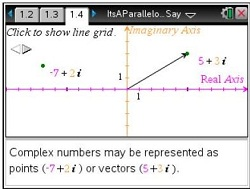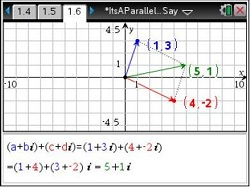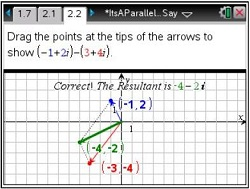# Activities

••• ##### Subject Area

• Math: Precalculus: Complex Numbers

• ##### Author9-12

30 Minutes

• ##### Device
•TI-Nspire™ CX/CX II
•TI-Nspire™ CX CAS/CX II CAS
• TI-Nspire™
• TI-Nspire™ CAS
• ##### Software

TI-Nspire™
TI-Nspire™ CAS

3.2

## It's a Parallelogram, You Say?

#### Activity Overview

Students represent complex numbers in the complex plane as points or vectors and display the sum and difference of two complex numbers as diagonals of the parallelograms they define.

#### Key Steps

•Students will explore the addition and subtraction of complex numbers in the complex coordinate plane using vector addition.

They are first presented with how to graph a complex number on a coordinate plane.

•Students explore of the concept of vector addition by grabbing and dragging the points at the end of the two bold vectors.

•Students are given addition and subtraction problems to solve for which a parallelogram has been provided. The student is to drag the tip of each vector to a point that represents the corresponding complex number, then use the resultant to determine the solution to the problem.